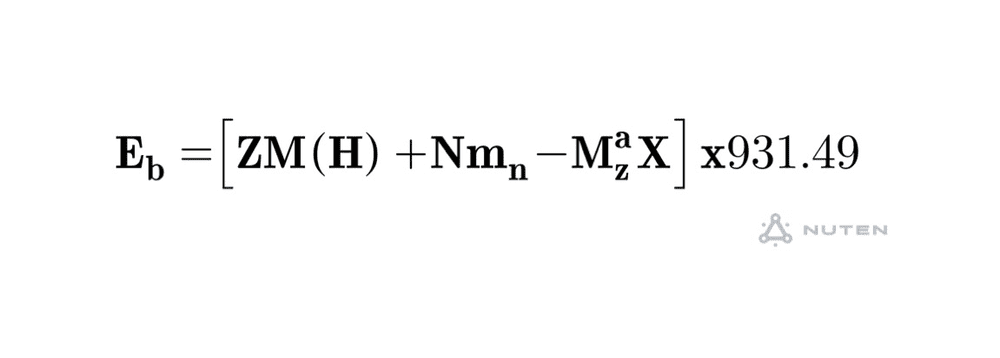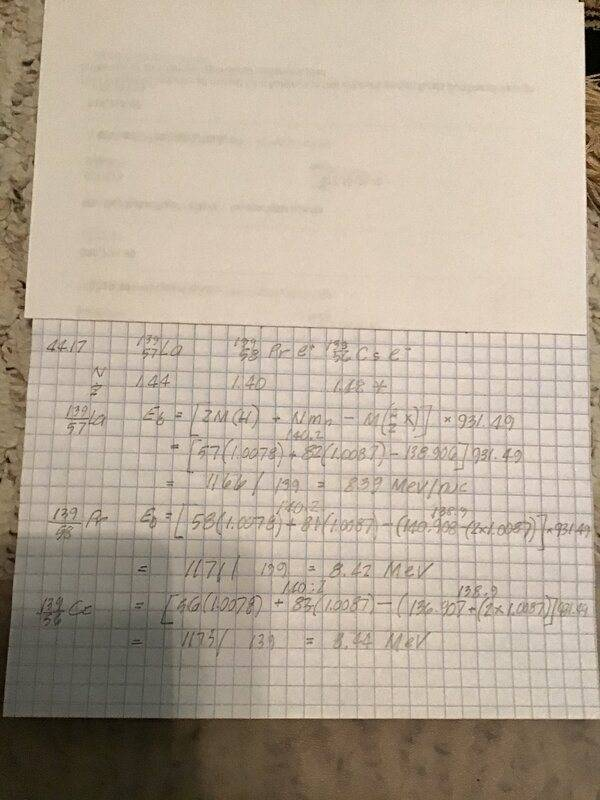# Isobar Binding Energy

• jjson775
In summary, isotopes with the same mass numbers are called isobars. The isotope 139/57 La is stable, while the radioactive isobar 139/59 Pr decays by e+ emission and the radioactive isobar 139/55 Cs decays by e- emission. The La isobar has the highest neutron to proton ratio, while the La isobar also has the greatest binding energy per nucleon. However, there may be discrepancies in atomic mass calculations due to the need for precision.

#### jjson775

Homework Statement
Nuclei having the same mass numbers are called isobars. The isotope 139/57 La is stable. A radioactive isobar 139/59 Pr decays by e+ emission. Another radioactive isobar 139/55 Cs, decays by e- emission. a) Which of these 3 isobars has the highest neutron to proton ratio? b) Which has the greatest binding energy per nucleon?
Relevant Equations
See belowjjson775 said:
Homework Statement:: Nuclei having the same mass numbers are called isobars. The isotope 139/57 La is stable. A radioactive isobar 139/59 Pr decays by e+ emission. Another radioactive isobar 139/55 Cs, decays by e- emission. a) Which of these 3 isobars has the highest neutron to proton ratio? b) Which has the greatest binding energy per nucleon?
Relevant Equations:: See below

View attachment 272898
View attachment 272899
How are you calculating the atomic masses of the isotopes? I just looked them up, and calculate that the La has the highest binding energy, as expected for the most stable.

139/59 Pr does not exist according to my table. So, to calculate the atomic mass to use in the binding formula, I used 140.908 for 141 Pr and subtracted the mass of 2 neutrons to give me an atomic mass of 138.9 for 139 Pr., as shown in the picture of my work. Apparently, my reasoning is wrong because the binding energy I get is too big.

jjson775 said:
139/59 Pr does not exist according to my table. So, to calculate the atomic mass to use in the binding formula, I used 140.908 for 141 Pr and subtracted the mass of 2 neutrons to give me an atomic mass of 138.9 for 139 Pr., as shown in the picture of my work. Apparently, my reasoning is wrong because the binding energy I get is too big.
At https://onlinelibrary.wiley.com/doi/pdf/10.1002/9783527618798.app2 it is given as 138.9089322.
The problem with your approximation method is that all three will have binding energies (correction: I mean, of course, atomic masses) close to 138.9, so precision is crucial.

Last edited:
Thanks. I found a reference with more precise atom masses.